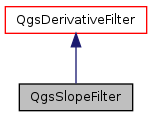QGIS API Documentation  2.8.2-Wien
QgsSlopeFilter Class Reference

Calculates slope values in a window of 3x3 cells based on first order derivatives in x- and y- directions. More...

`#include <qgsslopefilter.h>`

Inheritance diagram for QgsSlopeFilter:[legend]

## Public Member Functions

QgsSlopeFilter (const QString &inputFile, const QString &outputFile, const QString &outputFormat)
~QgsSlopeFilter ()
float processNineCellWindow (float *x11, float *x21, float *x31, float *x12, float *x22, float *x32, float *x13, float *x23, float *x33) override
Calculates output value from nine input values.Public Member Functions inherited from QgsDerivativeFilter
QgsDerivativeFilter (const QString &inputFile, const QString &outputFile, const QString &outputFormat)
virtual ~QgsDerivativeFilter ()Public Member Functions inherited from QgsNineCellFilter
QgsNineCellFilter (const QString &inputFile, const QString &outputFile, const QString &outputFormat)
Constructor that takes input file, output file and output format (GDAL string)
virtual ~QgsNineCellFilter ()
double cellSizeX () const
double cellSizeY () const
double inputNodataValue () const
double outputNodataValue () const
int processRaster (QProgressDialog *p)
Starts the calculation, reads from mInputFile and stores the result in mOutputFile.
void setCellSizeX (double size)
void setCellSizeY (double size)
void setInputNodataValue (double value)
void setOutputNodataValue (double value)
void setZFactor (double factor)
double zFactor () constProtected Member Functions inherited from QgsDerivativeFilter
float calcFirstDerX (float *x11, float *x21, float *x31, float *x12, float *x22, float *x32, float *x13, float *x23, float *x33)
Calculates the first order derivative in x-direction according to Horn (1981)
float calcFirstDerY (float *x11, float *x21, float *x31, float *x12, float *x22, float *x32, float *x13, float *x23, float *x33)
Calculates the first order derivative in y-direction according to Horn (1981)Protected Attributes inherited from QgsNineCellFilter
double mCellSizeX
double mCellSizeY
QString mInputFile
float mInputNodataValue
The nodata value of the input layer.
QString mOutputFile
QString mOutputFormat
float mOutputNodataValue
The nodata value of the output layer.
double mZFactor
Scale factor for z-value if x-/y- units are different to z-units (111120 for degree->meters and 370400 for degree->feet)

## Detailed Description

Calculates slope values in a window of 3x3 cells based on first order derivatives in x- and y- directions.

Definition at line 24 of file qgsslopefilter.h.

## Constructor & Destructor Documentation

 QgsSlopeFilter::QgsSlopeFilter ( const QString & inputFile, const QString & outputFile, const QString & outputFormat )

Definition at line 20 of file qgsslopefilter.cpp.

 QgsSlopeFilter::~QgsSlopeFilter ( )

Definition at line 26 of file qgsslopefilter.cpp.

## Member Function Documentation

 float QgsSlopeFilter::processNineCellWindow ( float * x11, float * x21, float * x31, float * x12, float * x22, float * x32, float * x13, float * x23, float * x33 )
overridevirtual

Calculates output value from nine input values.

The input values and the output value can be equal to the nodata value if not present or outside of the border. Must be implemented by subclasses

Implements QgsDerivativeFilter.

Definition at line 31 of file qgsslopefilter.cpp.

The documentation for this class was generated from the following files: